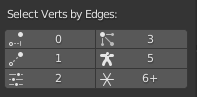# How to find vertices that don't belong to any face ? - python

Hi, how can I find vertices that don’t belong to any face (i.e. floating vertices not connected to anything else or only connected to one other vertex or connected to 2+ vertices but no face in between, etc) in Python?

There’s a “Select Loose Geometry” operator `bpy.ops.mesh.select_loose()` you can use. After running it you can loop through the geometry that was selected.

Then you can use the bmesh API to loop through vertices/edges/faces and see if they are selected:

EDIT:
Note that if you’re in vertex selection mode, you will only select loose vertices. So you won’t select a loose edge. If you’re in edge selection mode, you will select loose edges that don’t form a triangle.

1 Like

Ah` bpy.ops.mesh.select_loose()` also works for edges/faces too? let me try.

Yes, I actually edited my post right before your replies. You need to change selection modes.

If you switch to face select mode, loose_geometry will also select faces that are not connected to any other faces.

1 Like

Hello,
here is a similar request

and with @ckohl_art help
have this code

``````# Vertices with X edges connected - select
# https://devtalk.blender.org/t/select-all-verts-that-has-4-edges-connected/5328/11
class VERTX_OT_sel_vertx(Operator):
bl_idname = "vertx.sel_vertx"
bl_label = "Single Verteces"
bl_description = "Select vertexes with X connected edges"

list_values_imput: bpy.props.StringProperty(
name="List with values for imput",
description="Choose number of connected edges",
default="<= 1")

def execute(self, context):
bpy.ops.object.mode_set(mode='EDIT')
bpy.ops.mesh.select_mode(type="FACE")
bpy.ops.mesh.reveal()
bpy.ops.mesh.select_all(action='DESELECT')

aob = bpy.context.active_object

# 3D viewport should be in object mode
if not aob.mode == 'OBJECT' :
bpy.ops.object.editmode_toggle()

fmesh = bmesh.new()
fmesh.from_mesh(aob.data)

# Ensure bmesh internal index tables are fresh
fmesh.verts.ensure_lookup_table()
fmesh.edges.ensure_lookup_table()
fmesh.faces.ensure_lookup_table()

# cand: verts member of X edges and visible
cand = eval("[v for v in fmesh.verts if v.hide == False and len(v.link_edges) " + self.list_values_imput + "]")

pick = list()
# Select candidates with all X neighboring vertices also visible
for v in cand :
somehidden = False
somehidden = somehidden or e.other_vert(v).hide
if not somehidden :
v.select_set(True)
pick.append(v)
else :
v.select_set(False)

# Sync up bmesh and mesh
fmesh.to_mesh(aob.data)

bpy.ops.object.mode_set(mode='EDIT')
bpy.ops.mesh.select_mode(type="VERT")

#checking if verts is selected, then use Select Similar operator
me = bpy.context.object.data
bm = bmesh.from_edit_mesh(me)
for vert in bm.verts:
if vert.select:
bpy.ops.mesh.select_similar(type='EDGE', threshold=0.01)
break

return {'FINISHED'}
``````

And UI similar to this``````        layout.label(text="Select Verts by Edges:")
split = layout.split(align=True)
#row = layout.row(align=True)
col = split.column (align=True)
col.operator("vertx.sel_vertx", text = "0", icon = 'CON_LOCKTRACK').list_values_imput = "== 0"
col.operator("vertx.sel_vertx", text = "1", icon = 'CON_TRACKTO').list_values_imput = "== 1"
col.operator("vertx.sel_vertx", text = "2", icon = 'OPTIONS').list_values_imput = "== 2"
#row = layout.row(align=True)
col = split.column (align=True)
col.operator("vertx.sel_vertx", text = "3", icon = 'PARTICLES').list_values_imput = "== 3"
col.operator("vertx.sel_vertx", text = "5", icon = 'OUTLINER_OB_ARMATURE').list_values_imput = "== 5"
col.operator("vertx.sel_vertx", text = "6+", icon = 'SORTBYEXT').list_values_imput = ">= 6"
``````

Also you can use Select - Select All by Trait - Non Manifold (in vertex mode, and uncheck Boundaries)
And it will look like this

You can use a combination of `is_manifold`, `is_boundary`, and `is_wire` to determine the properties of a given vertex, edge, or face.

Here’s a simple script you can use to explore. While you’re in Edit mode on a mesh, click to select one vertex or edge or face and then run the script from the Script Editor. It will print to the console (Window > Toggle System Console).

``````import bpy
import bmesh

ctx = bpy.context
me = ctx.object.data
bm = bmesh.from_edit_mesh(me)

active = bm.select_history[-1]

print("----------")
print(active.index)
if active.is_manifold:
print("manifold")
if active.is_boundary:
print("boundary")
if active.is_wire:
print("wire")
``````

A floating vertex, not attached to anything, would return false for all 3.
A vertex that is attached to any number of edges but not to any face will return false for manifold and false for boundary but true for wire.

According to Blender:
A vertex is non-manifold if it meets any of the following conditions:
1: Loose - (has no edges/faces incident upon it).
2: Joins two distinct regions - (two pyramids joined at the tip).
3: Is part of an edge with more than 2 faces.
4: Is part of a wire edge.